1. Blog
2. Solar

# Comparing the Cost of Solar: Price Per Watt vs Levelized Cost Of Energy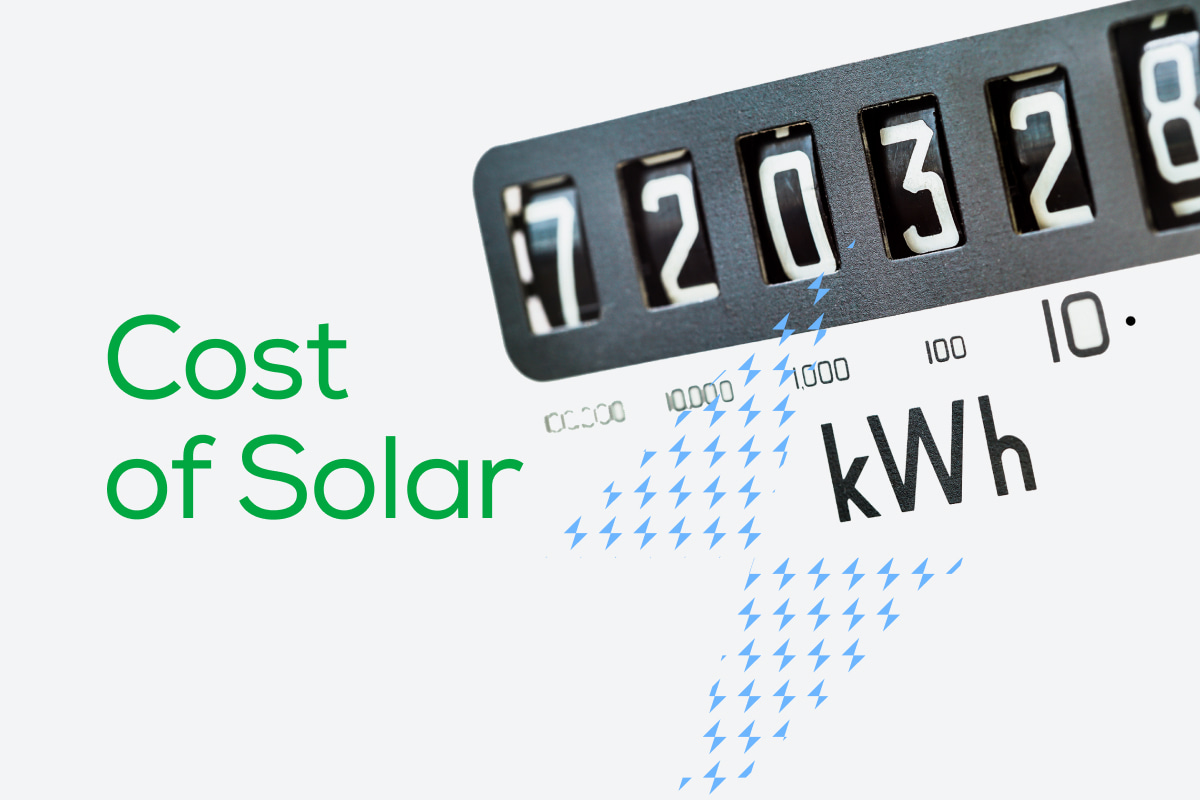One of the most common reasons that a homeowner considers installing solar panels is to save money on their electricity bills. The idea is that the price you pay for solar energy is less than the price you would have paid for that energy from your utility. For that to happen, solar shoppers need a clear understanding of what solar power costs, so they can make a well-informed decision that will maximize the return on their investment.

Two of the most valuable metrics for determining the upfront and long-term cost of a solar power system (and it's value) are price per watt (PPW) and the levelized cost of energy (LCOE). In this article, we will dive into the details of PPW and LCOE so you can learn what these terms mean, how to calculate them, and how they can help you make the right choice for your home.

## What Is Solar Price Per Watt?

When it comes to solar power, Price Per Watt (PPW) is the price that homeowners will pay for every watt of solar panel capacity installed. The price per watt is calculated by taking the net cost (price after incentives) of a solar panel system and dividing it by the total wattage of that system.

Keep in mind that solar power capacity is just a measurement of the maximum amount of power that system can generate in perfect conditions. It doesn’t mean that’s how much power the system will produce, because solar production is dependent on the amount and intensity of sun hours received.

Using PPW, a solar customer can compare solar panel pricing and the value of several solar power system quotes that may differ in wattage, system size, efficiency, and other factors.

To expand on a few concepts we just mentioned:

• Price per watt is measured in dollars per unit of power (\$/W).
• Net system cost is the total cost of installing your solar power system, after applying any rebates and incentives, including finance costs.
• The size of a solar panel system is typically shown in kilowatts (1kW = 1,000 watts), so multiply that number by 1,000 to get that number in watts (W).
• To calculate the price per watt, take the net system cost and divide it by the total wattage.

## How to Calculate the Solar Panel Price Per Watt

To calculate PPW, take the total price (after incentives) of the system you intend to buy, and divide it by the number of watts of generation capacity for the system: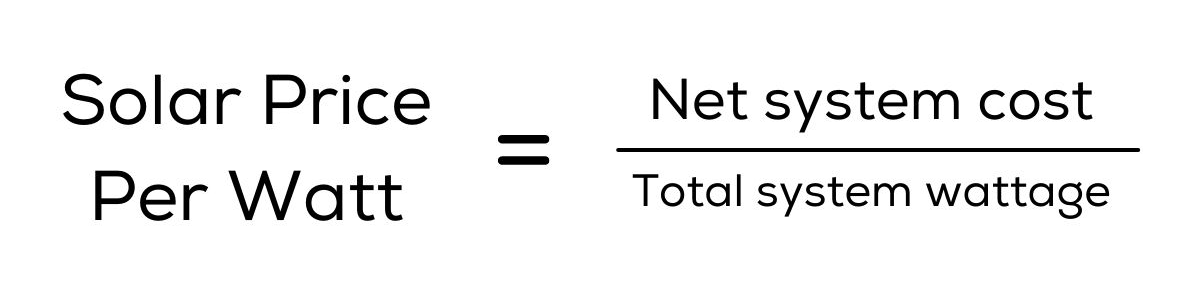For example, here’s the math for a 10 kW solar panel system with a quoted price of \$25,000: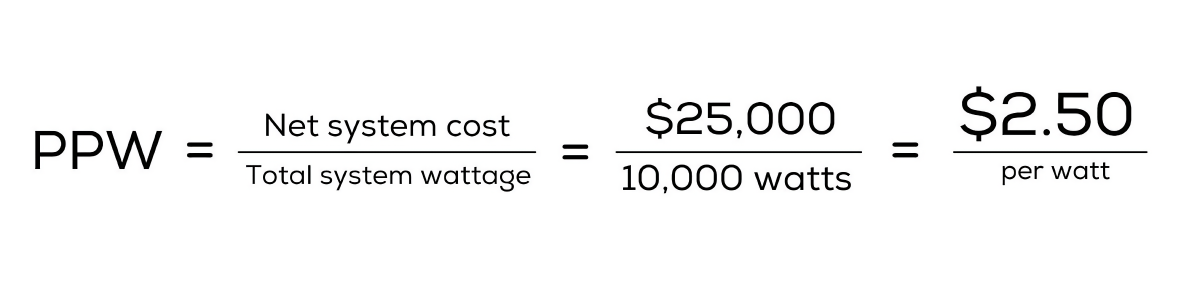In this example, the PPW is \$2.50 per watt.

## Why Is Price Per Watt Useful When Shopping for Solar Panels?

There are two basic ways to use price per watt when comparing quotes from different solar panel installers:

1. PPW helps compare one system against another, regardless of any difference in size.
2. PPW helps simplify what’s being paid for solar generation capacity, and what your payback period might be.

For example, imagine you want to compare two solar installations: One is an 8 kW solar panel system costing \$23,200, and the other is a 7 kW solar panel system costing \$20,300. How would you know which one offers more value for the money?

• 8 kW solar panel system has 8,000 watts = (\$23,200/8,000) = \$2.90/W
• 7 kW solar panel system has 7,000 watts = (\$20,300/7,000) = \$2.90/W

Thus, while they have different sizes and different costs, they both offer similar value in terms of the cost per unit of power output. If you only need 7 kW of solar power for your home, you could choose the cheaper system. But if you wanted to buy the larger system because you anticipate more energy consumption in the future, the actual PPW wouldn’t change.

## What Is Levelized Cost of Energy?

Levelized Cost of Energy (LCOE) is the average per-unit cost of any form of energy over the lifetime of the system. It's commonly used to compare the unit economics (the ratio of lifetime value to acquisition costs) of a variety of different energy-generating sources, such as natural gas, coal power plants, and green technologies.

Many solar companies use a version of LCOE to showcase the lifetime unit cost (\$/kWh) of electricity produced from a solar power system, compared to buying that same electricity from a traditional utility company. The goal is to show that the unit cost of electricity from the solar power system is cheaper than buying the same power from the utility, generating lifetime savings for the customer.

## How To Calculate the Levelized Cost of Energy Formula for Solar Panels

Historically, electricity rates have increased over time. When you install a solar power system, it’s like you’re locking in a specific rate for your electricity for the life of your solar panels, saving you from decades of rising retail rates.

This is what LCOE aims to prove, but calculating it is more complex than the PPW because it involves more data points and estimates of future production.

At a high level, LCOE considers:

1. The total price of the solar panel system after considering rebates and incentives.
2. The amount of daily sunlight the system is likely to get.
undefinedundefined
3. The overall efficiency of your solar system.
undefined
4. The size of your solar panel system.
5. The yearly rate of degradation of the system.
undefined

Finally, LCOE relies greatly on the installed system's geographic location and scale size. Hence, it's typically recorded as a range, not a precise value.

LCOE is calculated as: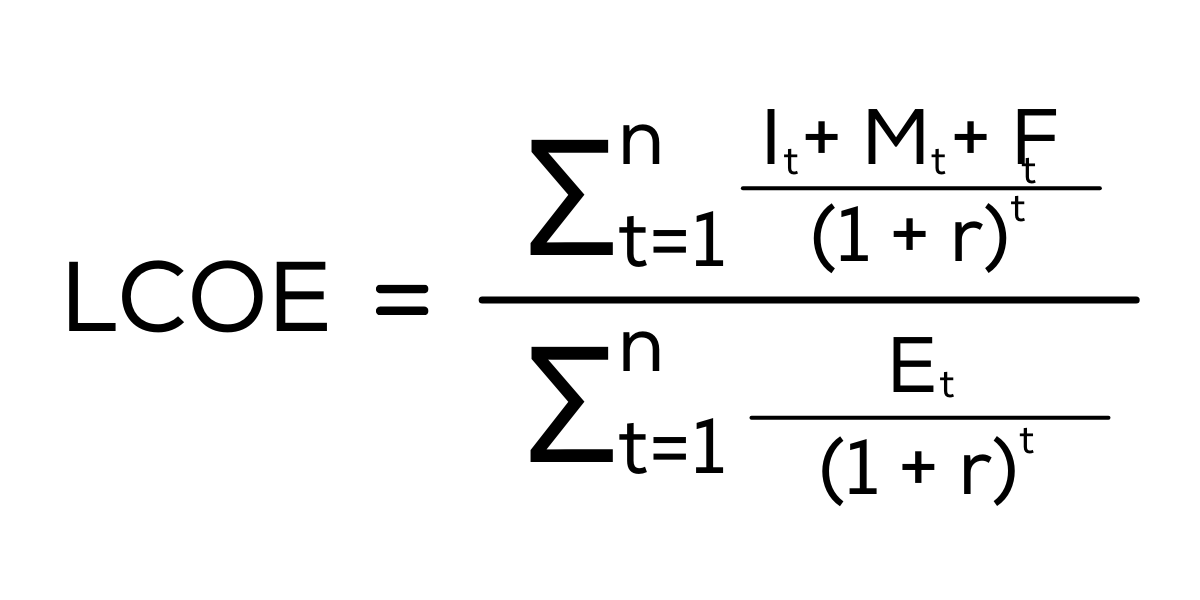In the Levelized Cost of Energy formula:

• It = Investment Expenditures (Capital Costs) In Year t (including financing)
• Mt = Operations and Maintenance Expenditures in Year t
• Ft = Fuel Expenditures (Fuel Costs) in Year t
• Et = Electricity Generation in Year t
• r = Discount Rate
• n = Life of the System

However, inputs like Discount Rate and Fuel Expenditures aren’t really relevant for most homeowners, and are better left to academic discussions about energy analysis and renewable energy technologies.

For home solar, we can simplify the LCOE calculation to just compare the amount of energy that a solar power system will produce over its lifetime, and the total cost of that system over its lifetime, including finance costs.

Stated a simpler way: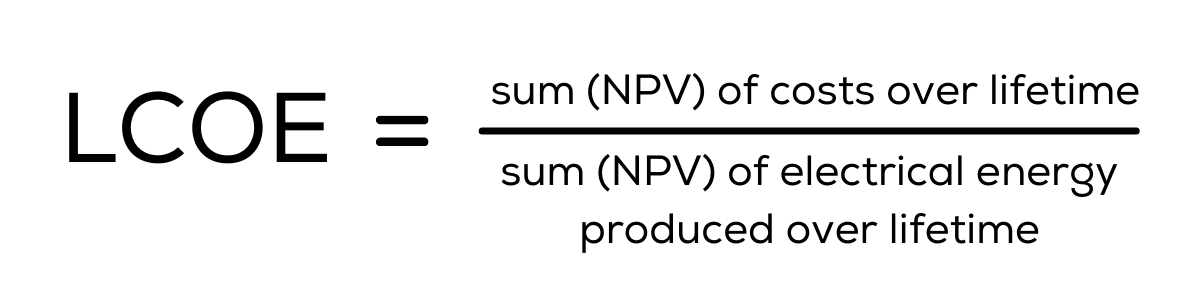The numerator (top number) in the formula includes the Net Present Value (NPV) of all costs that are often related to the long-term ownership of a solar panel system, including:

• Net system price
• Financing costs (the total amount of interest payments made over the lifetime of the system)
• Maintenance and service costs

Because LCOE looks at the net system price, it’s important to consider what your interest rate will be on the financial product you choose, and make sure you include the total cost (including interest payments) when looking at the lifetime \$/kWh. A system with a lower upfront cost that has a higher interest rate may end up having a worse LCOE, because those finance costs increase your lifetime costs, and eat into your savings.

The denominator (bottom number) includes the Net Present Value (NPV) of all generated energy produced throughout your solar panels’ lifetime. This formula factors in:

• Sunlight hours for your area and the time of day
• Capacity factor
• Nominal power

The denominator in the LCOE formula can be calculated as follows: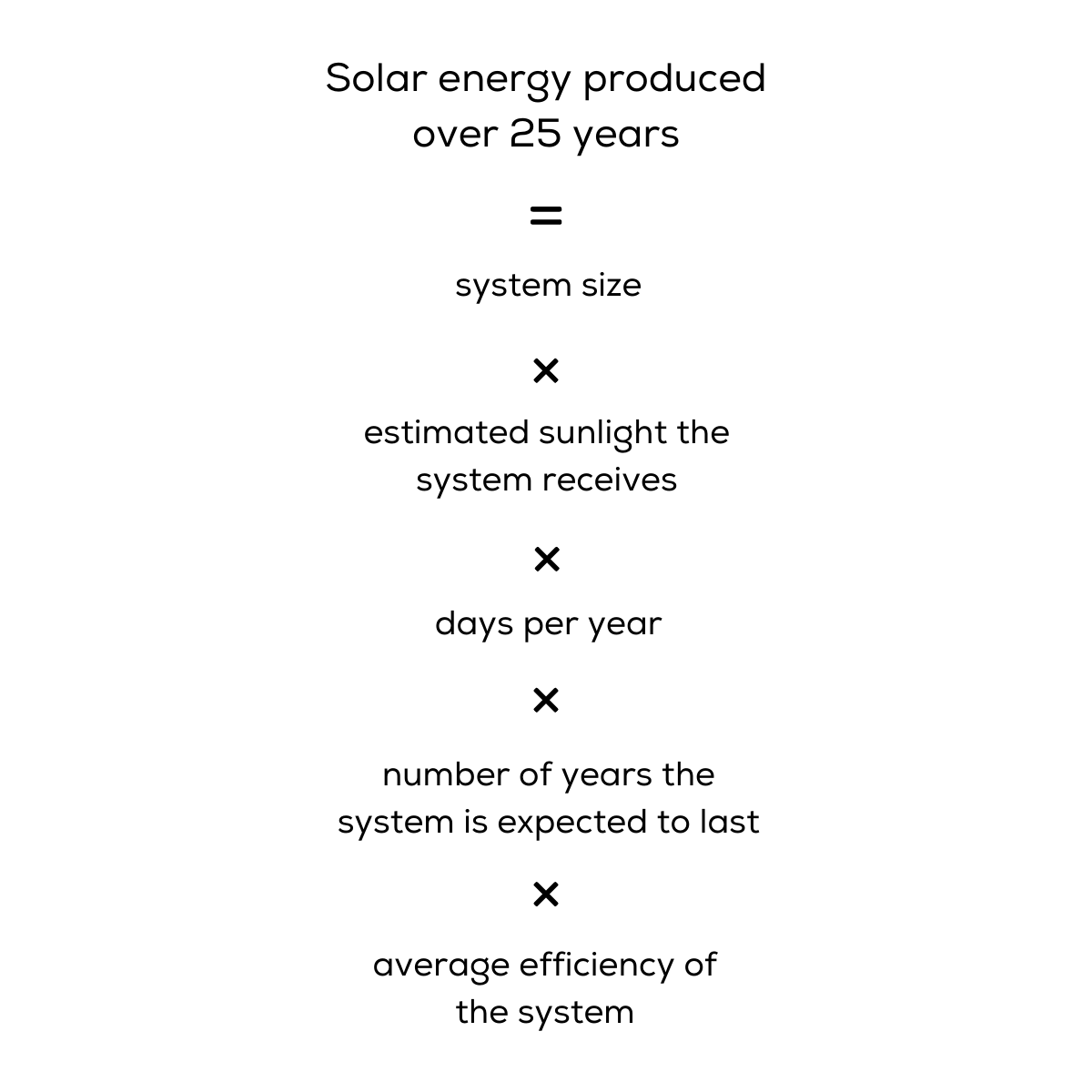## LCOE Example Calculation

Now, let’s calculate the LCOE in cents per kWh for a 10 kW system in Houston, TX.

To keep things simple, let’s say that the full cost of the system—which includes all upfront costs after incentives like the federal solar tax credit, plus finance charges and any ongoing maintenance costs—is \$20,000. That’s our numerator.

For our denominator, we can calculate it using the above formula: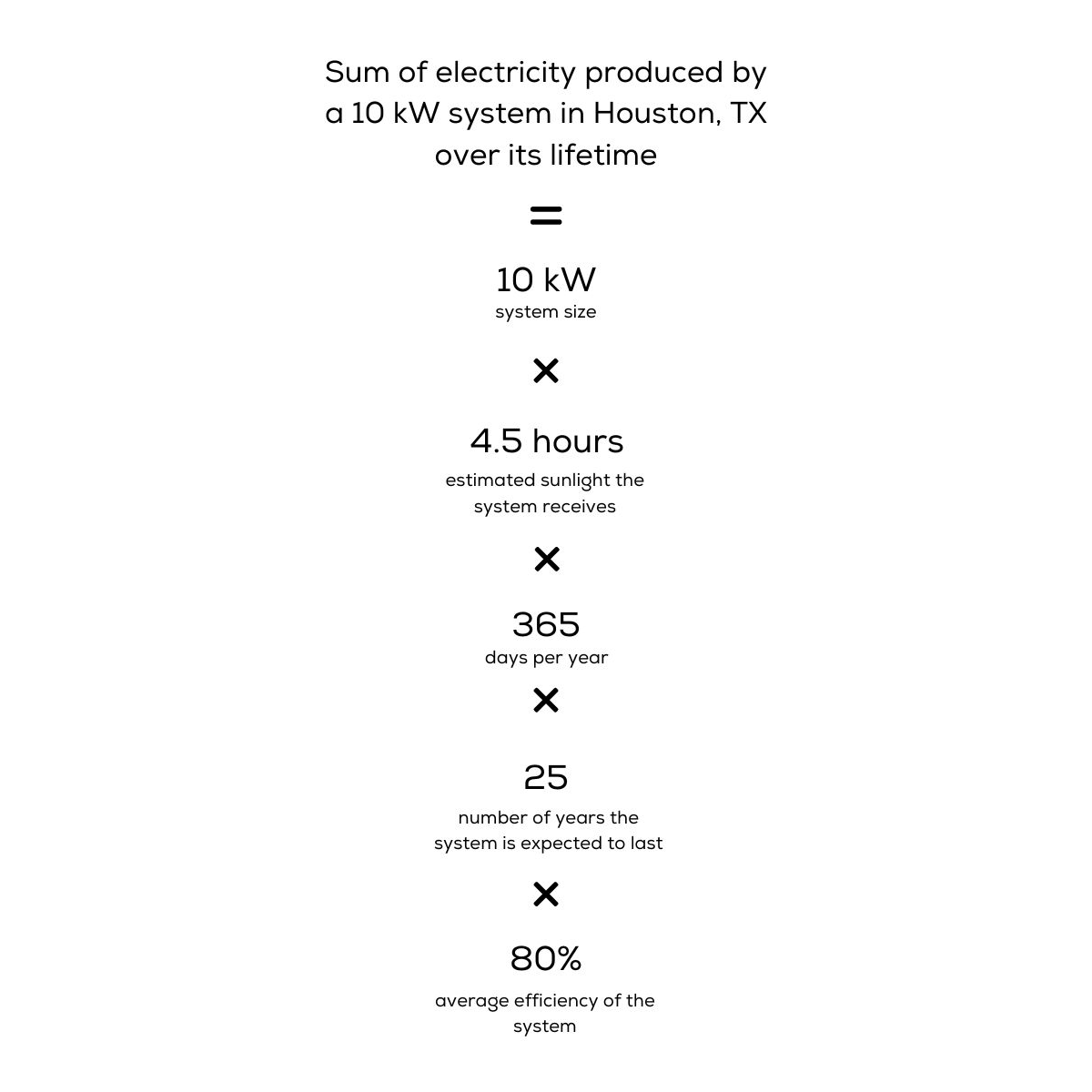That equals approximately 328,500 kWh.

After that, all you need to do is divide the total lifetime system cost (subtracting any solar incentives) by that number. It should look something like this: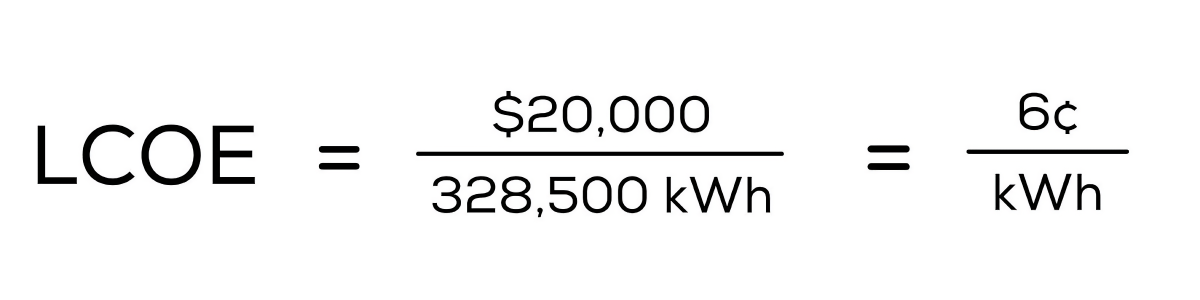At 6 cents per kilowatt-hour, that’s cheaper than the U.S. national average prices for residential electricity, which was 13.15 cents per kWh in 2020. (The national average for electricity prices is based on total utility revenue divided by kWh sold, so it will typically be higher than the cost you avoid by going solar, since it includes fixed charges and doesn't consider your utility’s net metering structure.)

And, since we know that electricity rates historically rise over time, the solar panel system in this theoretical calculation should continue to be cheaper than buying electricity from the utility company or retail electric provider.

Keep In Mind: This calculation is just an estimate!

You can use this equation to compare two similar solar panel systems before you install solar panels. If your solar systems produce more energy than expected, your cost per kWh will decrease. Conversely, if your solar energy system doesn't generate as much energy as expected, it will cost you more per kWh over the life of the system.

## Why Is LCOE Useful When Shopping for Solar Panels?

LCOE is a helpful tool for demonstrating the long-term feasibility of renewable energy, as it highlights the big-picture value of solar panels across 25-plus years. LCOE also shows how the cost per kWh of energy your system produces continues to go down over time, and the longer the system is in operation, the lower the cost of each kWh will be.

Further, LCOE enables you to measure the price of electricity produced by two different solar panels while other factors stay the same. That way, you will know which one will probably save you the most amount of money on your electricity bills.

For instance, the LCOE of a premium solar panel system could be 6.2¢/kWh, while another, less expensive system may have an LCOE of 7¢/kWh if it doesn’t last as long.

Lastly, because LCOE takes into account efficiency and expected sunlight, it allows you to compare similar systems in different locations. A 10 kW system that costs \$25,000 has the same PPW whether it’s installed in sunny Arizona or rainy Washington, but the expected sunlight those areas will receive means their LCOE will be drastically different. This can be helpful when comparing solar to various electricity rates by state.

In the end, the LCOE aims to figure out how much your solar power system will cost, after taking all factors into account, compared to the rate of your current electricity bill.

## The Difference Between Price Per Watt and the Levelized Cost of Energy

Price per watt (PPW) and levelized cost of energy (LCOE) are crucial considerations that offer different lenses into the value of solar panels:

• You can use PPW to compare solar panel system installation costs and solar power prices.
• You can use LCOE to compare the average per-unit cost of the electricity your solar panel will produce.
• PPW focuses on the upfront costs that people can understand more easily, as it uses large, round numbers.
• LCOE focuses on long-term costs and value, but it can be more challenging to understand since the formula is complex and the terms are more nebulous and based on assumptions.

## How To Compare the Cost of Solar

The price per watt and the levelized cost of energy are two crucial metrics for evaluating the cost of solar panels and return on investment of installing solar panels. Each provides a window of comparison that customers can use when evaluating solar panels:

• PPW looks at the upfront cost compared to the system size
• LCOE looks at the long-term value of the system's generation compared to the cost of the system

If you want to speak with a solar panel installer who can help you make sense of PPW and LCOE, Palmetto is here to help. Our experienced solar professionals will happily answer your questions.

You can get started today with our Free Solar Design and Savings Estimate Tool to get an instant quote for the cost of solar, and to learn how much you could save.

## See how much you can benefit bygoing solar with Palmetto

\$290
What's your monthly electric bill amount?